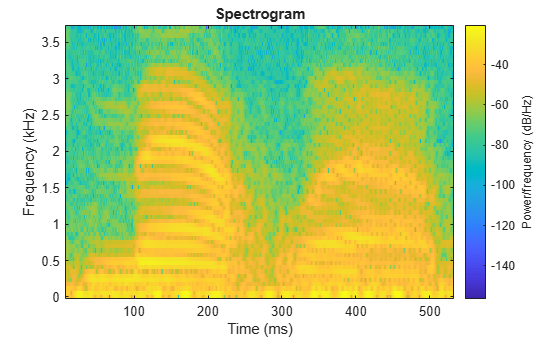# Complex Cepstrum — Fundamental Frequency Estimation

This example shows how to estimate a speaker's fundamental frequency using the complex cepstrum. The example also estimates the fundamental frequency using a zero-crossing method and compares the results.

Load the speech signal. The recording is of a woman saying "MATLAB". The sampling frequency is 7418 Hz. The following code loads the speech waveform, `mtlb`, and the sampling frequency, `Fs`, into the MATLAB® workspace.

`load mtlb`

Use the spectrogram to identify a voiced segment for analysis.

```segmentlen = 100; noverlap = 90; NFFT = 128; spectrogram(mtlb,segmentlen,noverlap,NFFT,Fs,'yaxis')```Extract the segment from 0.1 to 0.25 seconds for analysis. The extracted segment corresponds roughly to the first vowel, /æ/, in "MATLAB".

```dt = 1/Fs; I0 = round(0.1/dt); Iend = round(0.25/dt); x = mtlb(I0:Iend);```

Obtain the complex cepstrum.

`c = cceps(x);`

Select a time range between 2 and 10 ms, corresponding to a frequency range of approximately 100 to 500 Hz. Identify the tallest peak of the cepstrum in the selected range. Find the frequency corresponding to the peak. Use the peak as the estimate of the fundamental frequency.

```t = 0:dt:length(x)*dt-dt; trng = t(t>=2e-3 & t<=10e-3); crng = c(t>=2e-3 & t<=10e-3); [~,I] = max(crng); fprintf('Complex cepstrum F0 estimate is %3.2f Hz.\n',1/trng(I))```
```Complex cepstrum F0 estimate is 239.29 Hz. ```

Plot the cepstrum in the selected time range and overlay the peak.

```plot(trng*1e3,crng) xlabel('ms') hold on plot(trng(I)*1e3,crng(I),'o') hold off```Use a zero-crossing detector on a lowpass-filtered and rectified form of the vowel to estimate the fundamental frequency.

```[b0,a0] = butter(2,325/(Fs/2)); xin = abs(x); xin = filter(b0,a0,xin); xin = xin-mean(xin); x2 = zeros(length(xin),1); x2(1:length(x)-1) = xin(2:length(x)); zc = length(find((xin>0 & x2<0) | (xin<0 & x2>0))); F0 = 0.5*Fs*zc/length(x); fprintf('Zero-crossing F0 estimate is %3.2f Hz.\n',F0)```
```Zero-crossing F0 estimate is 233.27 Hz. ```

The estimate of the fundamental frequency obtained with the complex cepstrum is 239.29 Hz and the estimate with the zero-crossing detector is 233.27 Hz.